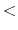Next: Targeted Molecular Dynamics (TMD) Up: User Defined Forces Previous: Rotating Constraints   Contents   Index

## Symmetry Restraints

Symmetry restraints are based on symmetrical relationships between monomers. Defined monomers are transformed to overlap and an average position for each atom is calculated. After the average structure is transformed back, a harmonic force is calculated which drives each monomer to the average.

• symmetryRestraintsAre symmetry restraints active?Acceptable Values: on or off
Default Value: off
Description: Should Symmetry constraining forces be applied to the system. If symmetry restraints are enabled, symmetryk* and symmetryFile must be defined in the input file as well. *See symmetryk entry for details.

• symmetryFirstFullStepFirst step to apply full harmonic forceAcceptable Values: Non-negative integer
Default Value: symmetryFirstStep
Description: Force constant symmetryk linearly increased from symmetryFirstStep to symmetryFirstFullStep

• symmetryLastFullStepLast step to apply full harmonic forceAcceptable Values: Non-negative integer
Default Value: symmetryLastStep
Description: Force constant symmetryk linearly decreased from symmetryLastFullStep to symmetryLastStep

• symmetrykConstant for harmonic restraining forcesAcceptable Values: Positive value
Description: Harmonic force constant. Scaled down by number of atoms in the monomer. If this setting is omitted, the value in the occupancy column of the pdb file specified by symmetrykFile will be used as the constant for that atom. This allows the user to specify the constant on a per-atom basis.

• symmetrykFilepdb containing per atom force constantsAcceptable Values: Path to pdb file
Description: pdb where the occupancy column specifies the per atom force constants. If using overlapping symmetry groups, you must include one additional symmetrykfile per symmetryFile

• symmetryScaleForcesScale symmetry restraints over timeAcceptable Values: on or off
Default Value: off
Description: If turned on, the harmonic force applied by the symmetry restraints will linearly evolve with each time step based on symmetryFirstFullStep and symmetryLastFullStep.

• symmetryFileFile for symmetry informationAcceptable Values: Path to PDB file
Description: Restrained atoms are those whose occupancy (O) is nonzero in the symmetry pdb file. The file must contain no more atoms than the structure file and those atoms present must have the exact same index as the structure file (i.e., the file may contain a truncated atom selection ``index'' but not an arbitrary selection). The value in the occupancy column represent the "symmetry group" the atom belongs to. These symmetry groups are used for denoting monomers of the same type. These groups will be transformed by the matrices in their own symmetryMatrixFile and averaged separetely from other groups. The designation in the occupancy column should be an integer value starting at 1 and proceeding in ascending order, mirroring the order of the corresponding matrix file within the configuration file (e.g. the first symmetryMatrixFile contains the matrices for symmetry group 1). The value in the atom's beta column represents its monomer designation. This should be an integer value starting at 1 and proceeding in ascending order, relative to the order of the corresponding transformation matrix found in the symmetry group's symmetryMatrixFile. If an atom is contained in more than one symmetry group, additional pdb files can be listed. These pdb files should follow the same rules as the first one (unique group and monomer identifiers in increasing order).

• symmetryMatrixFileFile for transformation matricesAcceptable Values: Path to matrix file
Description: The symmetryMatrixFile is a path to a file that contains a list of transformation matrices to make the monomers overlap. The file should contain one (and only one) matrix for each monomer in the order of monomer ID designated in the symmetryFile. Each symmetry group should have its own symmetryMatrixFile file containing only the matrices used by the monomers in that group. These should be formatted with spaces between columns and NO spaces between rows as follows:

1 0 0 0
0 1 0 0
0 0 1 0
0 0 0 1

with different matrices separated by a single blank line (and no line before the first or after the last matrix). This file is OPTIONAL. Leave this line out to have namd generate the transformations for you.

• symmetryFirstStepfirst symmetry restraint timestepAcceptable Values: Non-negative integer
Default Value: 0
Description:
• symmetryLastSteplast symmetry restraint timestepAcceptable Values: Positive integer
Default Value: infinity
Description: Symmetry restraints are applied only between symmetryFirstStep and symmetryLastStep. Use these settings with caution and ensure restraints are only being applied when necessary (e.g. not during equilibration).Next: Targeted Molecular Dynamics (TMD) Up: User Defined Forces Previous: Rotating Constraints   Contents   Index
http://www.ks.uiuc.edu/Research/namd/